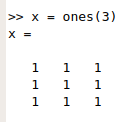# Initialize variables

## Initialize matrix with onesSometimes matrix multiply operations are hard to see and understand. With this small application done in C++ in Qt I try to show how a matrix multiplication of range equal three is done.LU factorization is a popular method to decompose a matrix as the product of a Lower triangle and an Upper triangle matrices. Lot of simulations perform the solving of a given system of linear equations. In general, the resolution of these systems is quite hard so matrix decomposition method is frequently used to subdivide the problem. As it's easier to solve a triangular matrix than a standard matrix, this is usually the first step on many simulations.A Huffman compressor is a compressor based on the Huffman Codification. This algorithm examines the text counting the frequences of each character. Then, it makes a new codification using that frequences. For example, in order to reduce the space, it`s easy to see that if we store 'C' letter 5 times as 0, 'A' letter 2 times as 10 and 'B' letter 3 times as 11 we achieve reducing the size compared with binary codification: